# A Look Back on 2018: Part 2

February 11, 2019
By

Want to share your content on R-bloggers? click here if you have a blog, or here if you don't.

Welcome to the second installment of Reproducible Finance 2019!

In the previous post, we looked back on the daily returns for several market sectors in 2018. Today, we’ll continue that theme and look at some summary statistics for 2018, and then extend out to previous years and different ways of visualizing our data. There’s not much heavy computation or even modeling today, but the goal is to lay some foundational code that we could use for different years or buckets of stocks, and to create some exploratory visualizations.

First, let’s load up our packages for the day.

``````library(tidyverse)
library(tidyquant)
library(riingo)
library(highcharter)``````

Next let’s grab our prices and returns for market sectors. We covered this in detail in the previous post and I won’t walk through it again, but here is the full code.

Note one change: last time, we imported data and calculated returns for just 2018. Today, I’ll set the start date to `start_date = "2007-12-29"` and import data for the 10 years from 2008 – 2018. That’s because, in addition to looking at summary statistics in just 2018, we will also look at some stats on a yearly basis from 2008 – 2018.

Here’s the code to import prices and calculate daily returns for our sector ETFs.

``````etf_ticker_sector <- tibble(
ticker = c("XLY", "XLP", "XLE",
"XLF", "XLV", "XLI", "XLB",
"XLK", "XLU", "XLRE",
"SPY"),
sector = c("Consumer Discretionary", "Consumer Staples", "Energy",
"Financials", "Health Care", "Industrials", "Materials",
"Information Technology", "Utilities", "Real Estate",
"Market")
)

sector_returns_2008_2018 <-
etf_ticker_sector %>%
pull(ticker) %>%
riingo_prices(.,
start_date = "2007-12-29",
end_date = "2018-12-31") %>%
mutate(date = ymd(date)) %>%
left_join(etf_ticker_sector, by = "ticker") %>%
group_by(sector) %>%
na.omit() ``````

Let’s take a quick peek at the first observation for each sector by using `slice(1)`, which will respect our `group_by()`.

``````sector_returns_2008_2018 %>%
group_by(sector) %>%
slice(1)``````
``````# A tibble: 11 x 4
# Groups:   sector 

1 Consumer Discretionary 2008-01-02     27.3     -0.0154
2 Consumer Staples       2008-01-02     21.1     -0.0143
3 Energy                 2008-01-02     62.5      0.00189
4 Financials             2008-01-02     18.6     -0.0199
5 Health Care            2008-01-02     28.8     -0.0105
6 Industrials            2008-01-02     30.4     -0.0167
7 Information Technology 2008-01-02     21.8     -0.0205
8 Market                 2008-01-02    115.      -0.00879
9 Materials              2008-01-02     32.2     -0.00964
10 Real Estate            2015-10-09     26.7     -0.00166
11 Utilities              2008-01-02     27.8     -0.00569``````

This looks good, but I’d like to confirm that we successfully imported prices and calculated returns for each year and for each sector, meaning I want `group_by(year, sector)` and then `slice(1)`. Problem is: there’s not currently a column called `year`.

We can fix that by separating the date column into `year` and `month` with the incredibly useful `separate()` function. We will run `separate(date, c("year", "month"), sep = "-", remove = FALSE)`. I use `remove = FALSE` because I want to keep the `date` column.

It’s not necessary, but for ease of viewing in this post, I’ll peek at just sectors that contain the word “Consumer”, by calling `filter(sector, str_detect("Consumer"))`.

``````sector_returns_2008_2018 %>%
separate(date, c("year", "month"), sep = "-", remove = FALSE) %>%
group_by(year, sector) %>%
slice(1) %>%
filter(str_detect(sector, "Consumer"))``````
``````# A tibble: 22 x 6
# Groups:   year, sector 
sector                 date       year  month adjClose daily_return

1 Consumer Discretionary 2008-01-02 2008  01        27.3    -0.0154
2 Consumer Staples       2008-01-02 2008  01        21.1    -0.0143
3 Consumer Discretionary 2009-01-02 2009  01        19.5     0.0489
4 Consumer Staples       2009-01-02 2009  01        18.5     0.0141
5 Consumer Discretionary 2010-01-04 2010  01        26.3     0.00770
6 Consumer Staples       2010-01-04 2010  01        20.9     0.00753
7 Consumer Discretionary 2011-01-03 2011  01        33.7     0.0117
8 Consumer Staples       2011-01-03 2011  01        23.6     0.00136
9 Consumer Discretionary 2012-01-03 2012  01        35.6     0.00842
10 Consumer Staples       2012-01-03 2012  01        26.9    -0.000924
# … with 12 more rows``````

OK, we’ve confirmed that we have prices and returns for our sectors for each year. Those new `month` and `year` columns will come in handy later, so let’s go ahead and save them.

``````sector_returns_2008_2018_year_mon <-
sector_returns_2008_2018 %>%
separate(date, c("year", "month"), sep = "-", remove = FALSE) %>%
group_by(year, sector)``````

We’re going to look back on several summary statistics for 2018 first: mean daily return, standard deviation, skewness, and kurtosis of daily returns. We will use the `summarise()` function and then `filter(year == "2018")` to get our stats for just 2018.

``````sector_returns_2008_2018_year_mon %>%
summarise(avg = mean(daily_return),
stddev = sd(daily_return),
skew = skewness(daily_return),
kurt = kurtosis(daily_return)) %>%
filter(year == "2018")``````
``````# A tibble: 11 x 6
# Groups:   year 
year  sector                        avg  stddev   skew  kurt

1 2018  Consumer Discretionary  0.0000629 0.0122  -0.199 2.61
2 2018  Consumer Staples       -0.000335  0.00915 -0.664 1.54
3 2018  Energy                 -0.000801  0.0140  -0.294 1.58
4 2018  Financials             -0.000557  0.0124  -0.675 2.47
5 2018  Health Care             0.000243  0.0111  -0.604 2.31
6 2018  Industrials            -0.000566  0.0120  -0.717 2.08
7 2018  Information Technology -0.0000667 0.0147  -0.336 1.82
8 2018  Market                 -0.000186  0.0108  -0.479 3.18
9 2018  Materials              -0.000641  0.0121  -0.210 0.861
10 2018  Real Estate            -0.0000957 0.0103  -0.548 1.41
11 2018  Utilities               0.000153  0.00956 -0.621 1.82 ``````

We can build off that code flow to select just the years 2014 and 2015 with `filter(year %in% c("2014", "2015")` and, say, the energy sector with `str_detect(sector, "Energy")`.

``````sector_returns_2008_2018_year_mon %>%
summarise(avg = mean(daily_return),
stddev = sd(daily_return),
skew = skewness(daily_return),
kurt = kurtosis(daily_return)) %>%
filter(year %in% c("2014", "2015") &
str_detect(sector, "Energy"))``````
``````# A tibble: 2 x 6
# Groups:   year 
year  sector       avg stddev    skew  kurt

1 2014  Energy -0.000361 0.0117 -0.891  4.43
2 2015  Energy -0.000959 0.0157  0.0157 0.813``````

Think about how that code flow might be useful in a Shiny application, where we let the end user choose a sector, a year, and possibly which summary stats to calculate and display.

Now let’s do some visualizing.

We’ll start with a column chart, where the height is equal to the sector skewness for the chosen year.

``````sector_returns_2008_2018_year_mon %>%
summarise(avg = mean(daily_return),
stddev = sd(daily_return),
skew = skewness(daily_return),
kurt = kurtosis(daily_return)) %>%
filter(year == "2018") %>%
ggplot(aes(x = sector, y = skew, fill = sector)) +
geom_col(width = .3) +
ylim(-1,1) +
theme(axis.text.x = element_text(angle = 90, vjust = 1, hjust=1))``````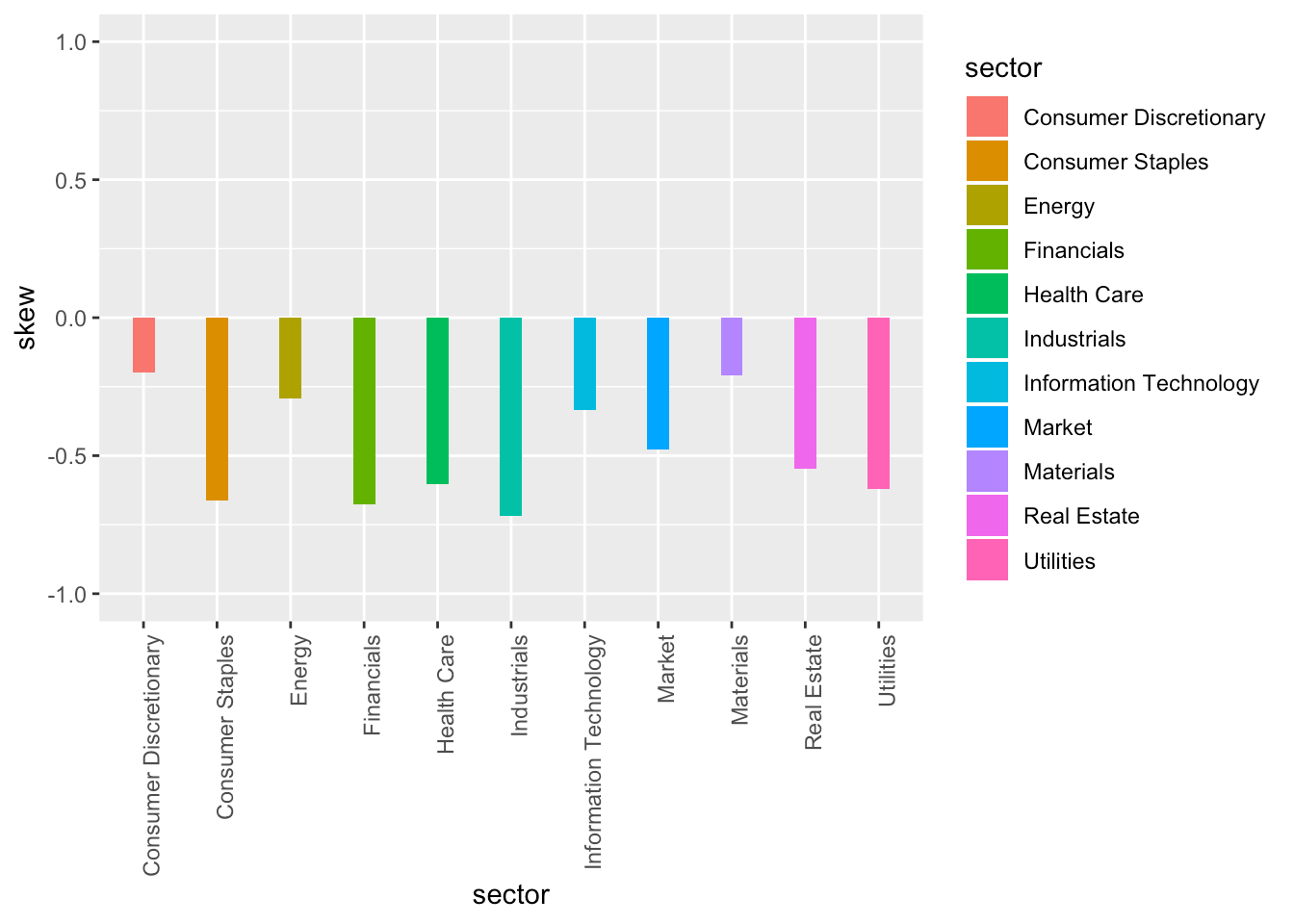Here’s the same exact data, except we’ll use a scatter plot where the height of each point is the skewness.

``````sector_returns_2008_2018_year_mon %>%
summarise(avg = mean(daily_return),
stddev = sd(daily_return),
skew = skewness(daily_return),
kurt = kurtosis(daily_return)) %>%
filter(year == "2018") %>%
ggplot(aes(x = sector, y = skew, color = sector)) +
geom_point(size = .8) +
ylim(-1, 1) +
theme(axis.text.x = element_text(angle = 90, vjust = 1, hjust=1))``````For both of the charts above, we could change our `filter(year == ...)` to choose a different year and build a new chart, but instead let’s comment out the year filter altogether, meaning we will chart all years, and then call `facet_wrap(~year)`.

``````sector_returns_2008_2018_year_mon %>%
summarise(avg = mean(daily_return),
stddev = sd(daily_return),
skew = skewness(daily_return),
kurt = kurtosis(daily_return)) %>%
# filter(year == "2018") %>%
ggplot(aes(x = sector, y = skew, fill = sector)) +
geom_col(width = .5) +
ylim(-1, 1) +
theme(axis.text.x = element_text(angle = 90, vjust = 1, hjust=1)) +
facet_wrap(~year)``````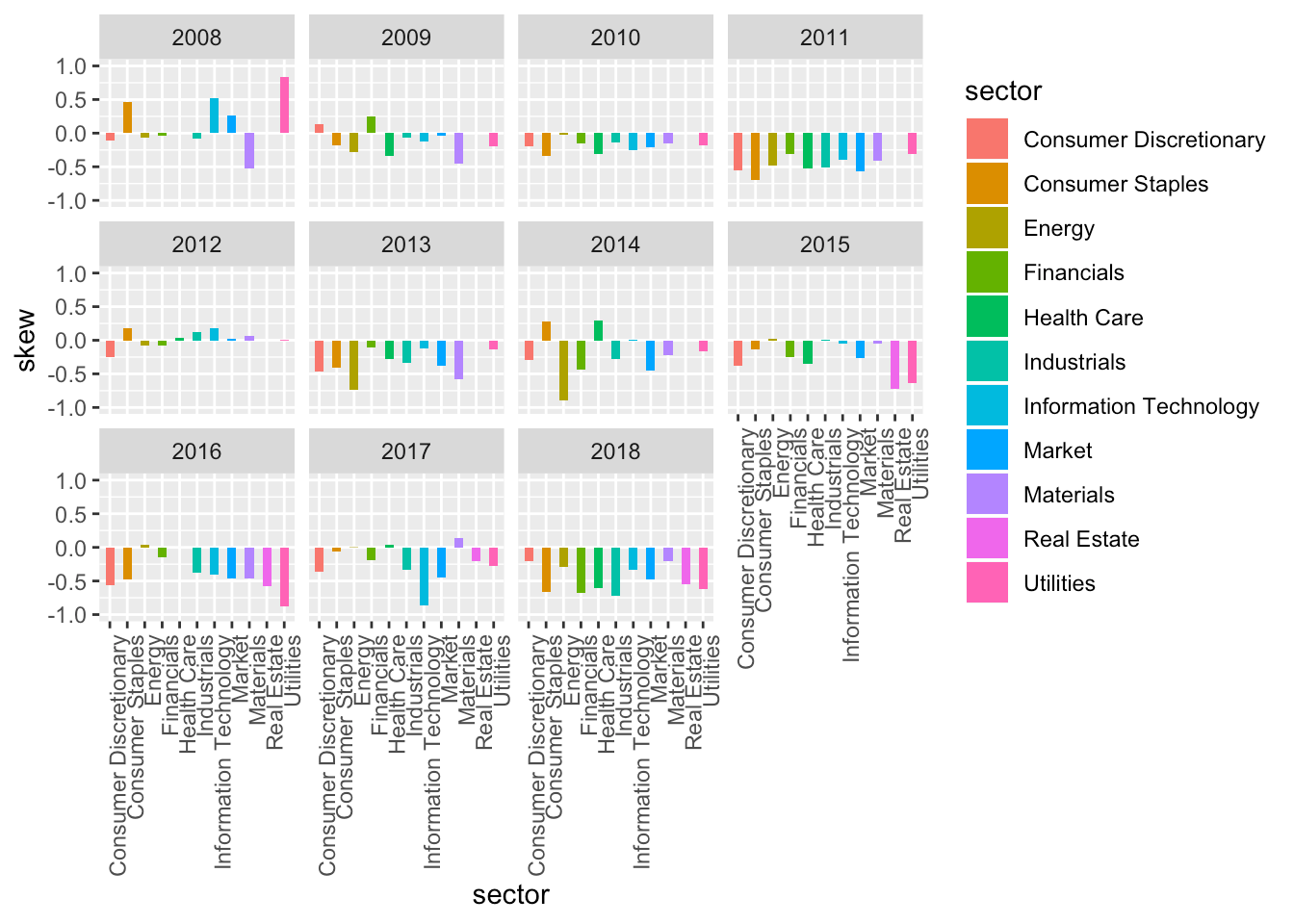This post was originally going to be focused on standard deviation, and not skewness, but there was recently an excellent piece on the Think New Found blog that discusses skewness and its importance as a risk measure. Definitely worth a read for the risk-return obsessed amongst us. For an R code reference, we covered skewness extensively in this previous blog post, and there’s bare code for the calculations on the Reproducible Finance site here.

Those ggplots are nice, but let’s take a quick look at how we might do this with `highcharter`.

``````sector_returns_2008_2018_year_mon %>%
summarise(avg = mean(daily_return),
stddev = sd(daily_return),
skew = skewness(daily_return),
kurt = kurtosis(daily_return)) %>%
filter(year == "2018") %>%
hchart(.,
type = 'column',
hcaes(y = skew,
x = sector,
group = sector)) %>%
hc_title(text = "2018 Sector Skew") %>%
hc_subtitle(text = "by sector") %>%
hc_xAxis(title = list(text = "")) %>%
pointFormat = "skewness: {point.y: .4f}%
mean return: {point.avg: .4f}") %>%
hc_yAxis(labels = list(format = "{value}%")) %>%
hc_exporting(enabled = TRUE) %>%
hc_legend(enabled = FALSE)``````

Hover on the bars and notice that we included the mean return for each sector as well. That’s the beauty of `highcharter`: we can easily add more data in the tooltip using the `hc_tooltip()` function. Those skews look pretty daunting, but that’s down to the scale of the y-axis of this chart, which defaults to a max of 0 and a minimum of .8%. Let’s coerce it to max of 1 and a min of -1, which is a rough boundary for where we are comfortable with skewness.

``````sector_returns_2008_2018_year_mon %>%
summarise(avg = mean(daily_return),
stddev = sd(daily_return),
skew = skewness(daily_return),
kurt = kurtosis(daily_return)) %>%
filter(year == "2018") %>%
hchart(.,
type = 'column',
hcaes(y = skew,
x = sector,
group = sector)) %>%
hc_title(text = "2018 Skew by Sector") %>%
hc_xAxis(title = list(text = "")) %>%
pointFormat = "skewness: {point.y: .4f}%
mean return: {point.avg: .4f}") %>%
hc_yAxis(labels = list(format = "{value}%"),
min = -1,
max =1) %>%
hc_exporting(enabled = TRUE) %>%
hc_legend(enabled = FALSE)``````

Let’s explore one more piece of data. After breaking up the date into year and month and looking at the daily returns and skewness, I got to wondering if the minimum daily return for each sector tended to fall in a certain month. There’s no reason it should, but it seems like a trend we might want to parse, or at least have thought about in case we need it.

My first instinct was to use `summarise()` and get the minimum daily return for each year-sector pair.

``````sector_returns_2008_2018_year_mon %>%
summarise(min_ret = min(daily_return)) %>%
``````# A tibble: 6 x 3
# Groups:   year 
year  sector                 min_ret

1 2008  Consumer Discretionary -0.116
2 2008  Consumer Staples       -0.0568
3 2008  Energy                 -0.160
4 2008  Financials             -0.182
5 2008  Health Care            -0.0687
6 2008  Industrials            -0.0947``````

The problem with that flow is that our `month` got deleted and we would like to preserve that for charting. We’re better off to `filter()` by the `min(daily_return)`.

``````sector_returns_2008_2018_year_mon %>%
filter(daily_return == min(daily_return)) %>%
group_by(sector) %>%
filter(year == "2008") %>%
``````# A tibble: 6 x 4
# Groups:   sector 
sector                 year  month daily_return

1 Consumer Discretionary 2008  10         -0.116
2 Consumer Staples       2008  12         -0.0568
3 Energy                 2008  10         -0.160
4 Financials             2008  12         -0.182
5 Health Care            2008  10         -0.0687
6 Industrials            2008  10         -0.0947``````

That’s giving us the same end data for the minimum daily return, but it’s also preserving the `month` column.

Let’s take a quick look to see if any months jump out as frequent holders of the minimum daily return. Note that we’ll need to `ungroup()` the data before running `count(month)`.

``````sector_returns_2008_2018_year_mon %>%
filter(daily_return == min(daily_return)) %>%
ungroup() %>%
count(month) ``````
``````# A tibble: 11 x 2
month     n

1 01        6
2 02       18
3 03        4
4 04        4
5 05       14
6 06       22
7 08       23
8 09        3
9 10        9
10 11        3
11 12        8``````

Hmmm, months 5, 6 and 8 jump out a bit. Let’s translate those to their actual names using `mutate(month = month(date, label = TRUE, abbr = FALSE))`.

``````sector_returns_2008_2018_year_mon %>%
filter(daily_return == min(daily_return)) %>%
mutate(month = month(date, label = TRUE, abbr = FALSE)) %>%
ungroup() %>%
count(month)``````
``````# A tibble: 11 x 2
month         n

1 January       6
2 February     18
3 March         4
4 April         4
5 May          14
6 June         22
7 August       23
8 September     3
9 October       9
10 November      3
11 December      8``````

Visualizing these monthly tendencies was a bit more involved than I had anticipated, and that usually means I’ve missed a simpler solution somewhere, but I’ll post my brute force insanity in case it’s helpful to others.

I want to create a chart that looks like this, with months on the x-axis and the minimum daily return for each sector on the y-axis, almost as if we’re trying to see if the minimum daily returns tend to cluster in any months.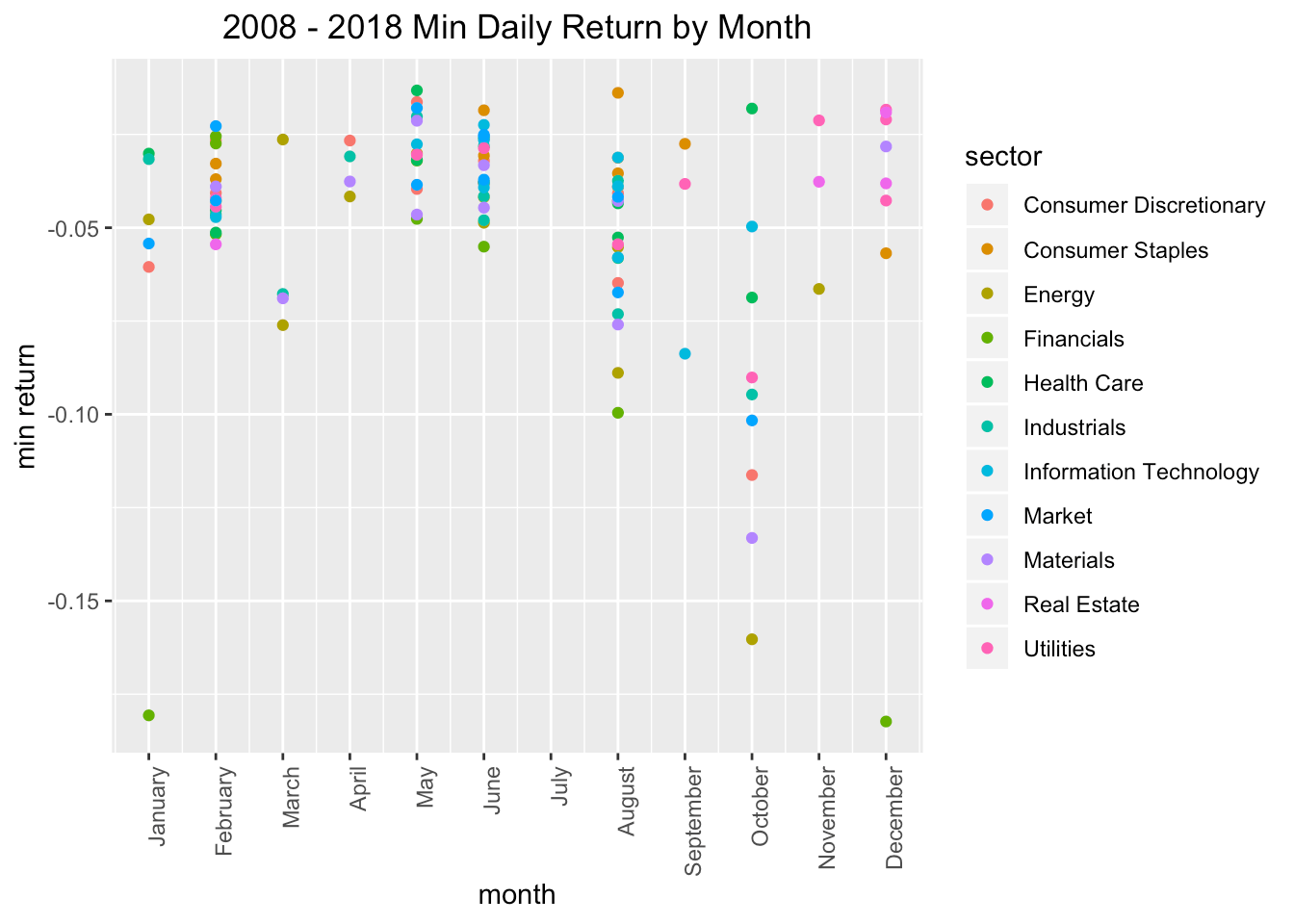To create that chart, I want the names of the months on the x-axis, but also in the correct order. If we coerce the numbers to month names ahead of charting, `ggplot` will put them in alphabetical order, which is not what we want.

To solve that problem, I first created a vector of months.

``````months <-
sector_returns_2008_2018_year_mon %>%
mutate(months = month(date, label = TRUE, abbr = FALSE)) %>%
pull() %>%
levels() %>%
as.character()

months``````
``````  "January"   "February"  "March"     "April"     "May"
 "June"      "July"      "August"    "September" "October"
 "November"  "December" ``````

Next, comes our usual flow from the sector returns to `ggplot`, but first we coerce the `month` column with `as.numeric()` (when we used `separate()` before, it created a character column). Then we put month on the x-axis with `ggplot(aes(x = month...))`. To create the proper labels, we use `scale_x_continuous( breaks = c(1, 2, 3, 4, 5, 6, 7, 8, 9, 10, 11, 12), labels = months)` to add 12 breaks and label them with our `months` vector that we created above.

``````  sector_returns_2008_2018_year_mon %>%
filter(daily_return == min(daily_return)) %>%
mutate(month = as.numeric(month)) %>%
ggplot(aes(x = month, y = daily_return, color = sector)) +
geom_point() +
scale_x_continuous(breaks = c(1, 2, 3, 4, 5, 6, 7, 8, 9, 10, 11, 12),
labels = months) +
labs(y = "min return", title = "2008 - 2018 Min Returns by Month") +
theme(axis.text.x = element_text(angle = 90, hjust = 1),
plot.title = element_text(hjust = 0.5)) ``````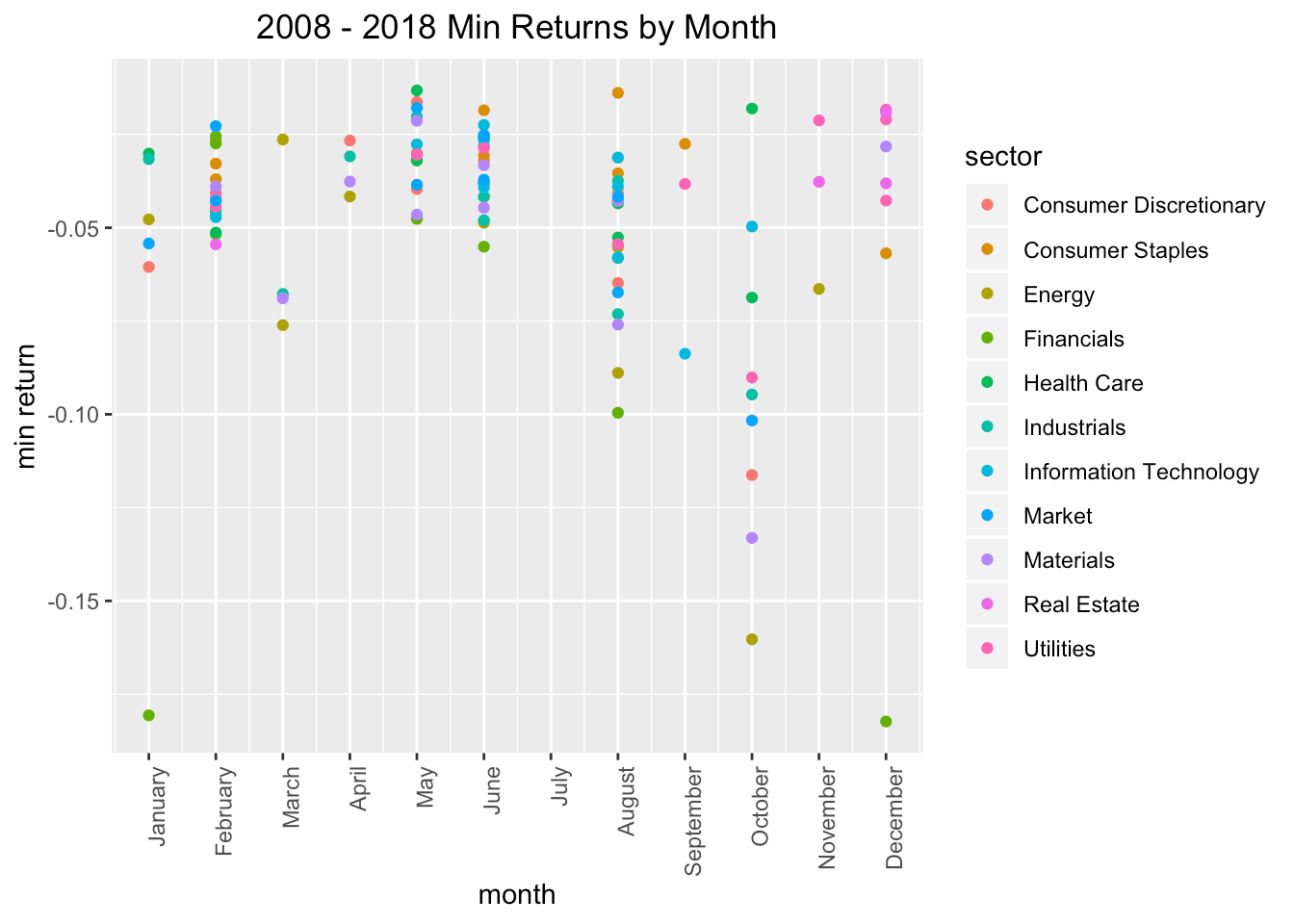We can facet by sector if we want to break this into pieces.

``````  sector_returns_2008_2018_year_mon %>%
filter(daily_return == min(daily_return)) %>%
mutate(month = as.numeric(month)) %>%
ggplot(aes(x = month, y = daily_return, color = sector)) +
geom_point() +
scale_x_continuous(breaks = c(1, 2, 3, 4, 5, 6, 7, 8, 9, 10, 11, 12),
labels = months)  +
labs(y = "min return", title = "2008 - 2018 Min Returns by Month") +
theme(axis.text.x = element_text(angle = 90, hjust = 1),
plot.title = element_text(hjust = 0.5)) +
facet_wrap(~sector)``````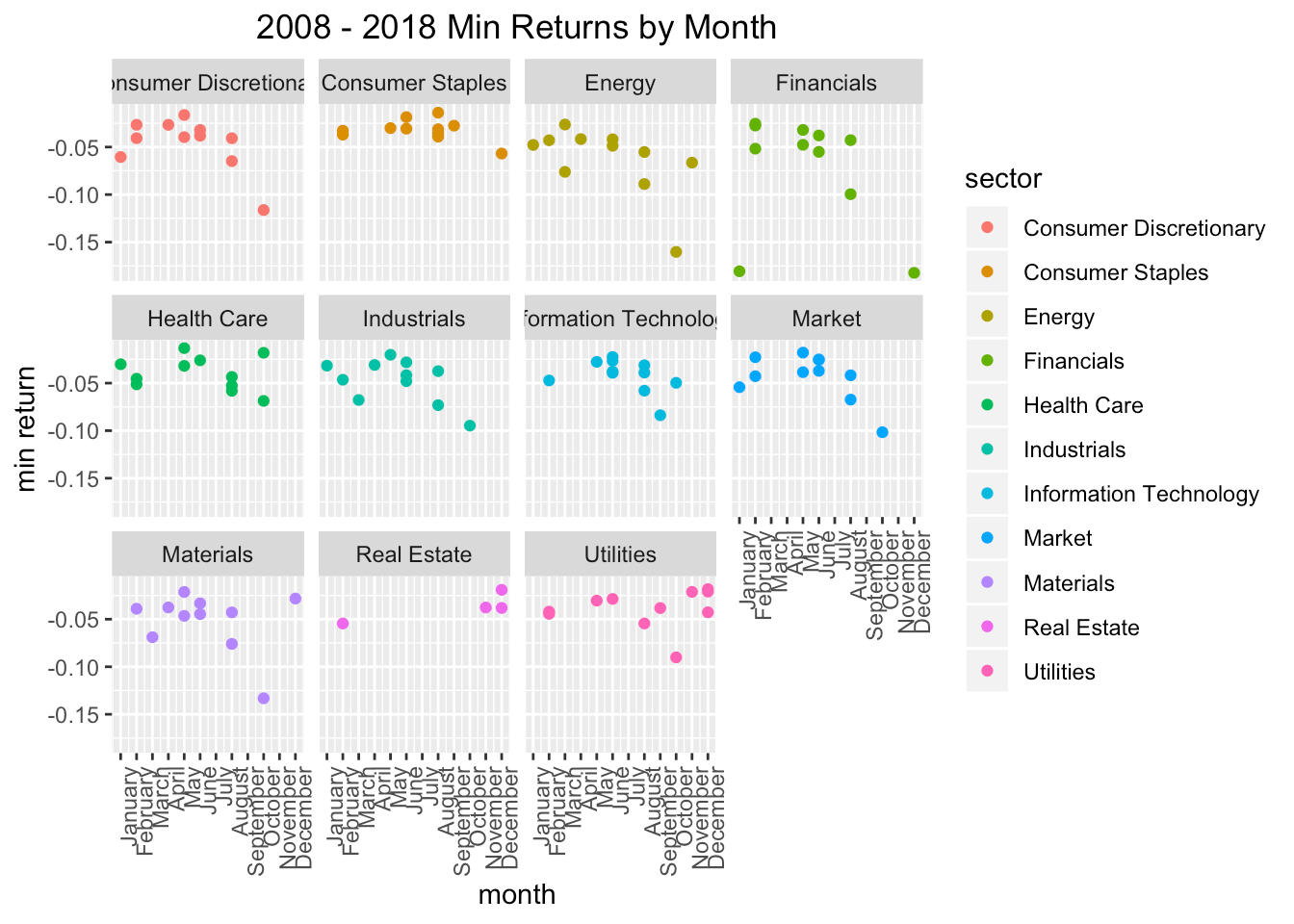Or we can facet by year.

``````sector_returns_2008_2018_year_mon %>%
filter(daily_return == min(daily_return)) %>%
mutate(month = as.numeric(month)) %>%
ggplot(aes(x = month, y = daily_return, color = sector)) +
geom_point() +
scale_x_continuous(breaks = c(1, 2, 3, 4, 5, 6, 7, 8, 9, 10, 11, 12),
labels = months)  +
labs(y = "min return", title = "2008 - 2018 Min Returns by Month") +
theme(axis.text.x = element_text(angle = 90, hjust = 1),
plot.title = element_text(hjust = 0.5)) +
facet_wrap(~year)``````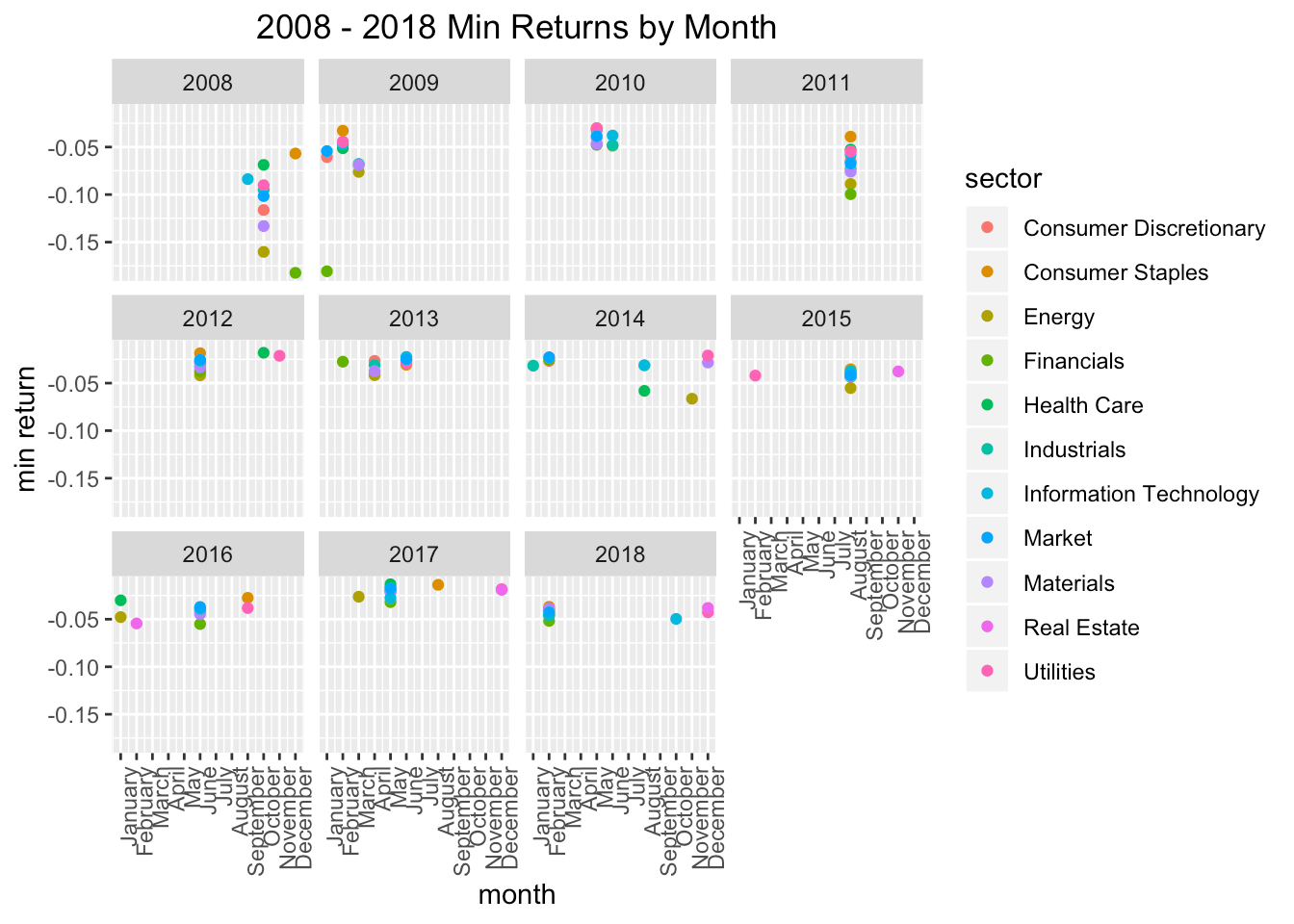Interesting to see that in 2011, each of our sectors had their minimum daily return in the same month.

That’s all for today. Thanks for reading and see you next time!

R-bloggers.com offers daily e-mail updates about R news and tutorials about learning R and many other topics. Click here if you're looking to post or find an R/data-science job.
Want to share your content on R-bloggers? click here if you have a blog, or here if you don't.